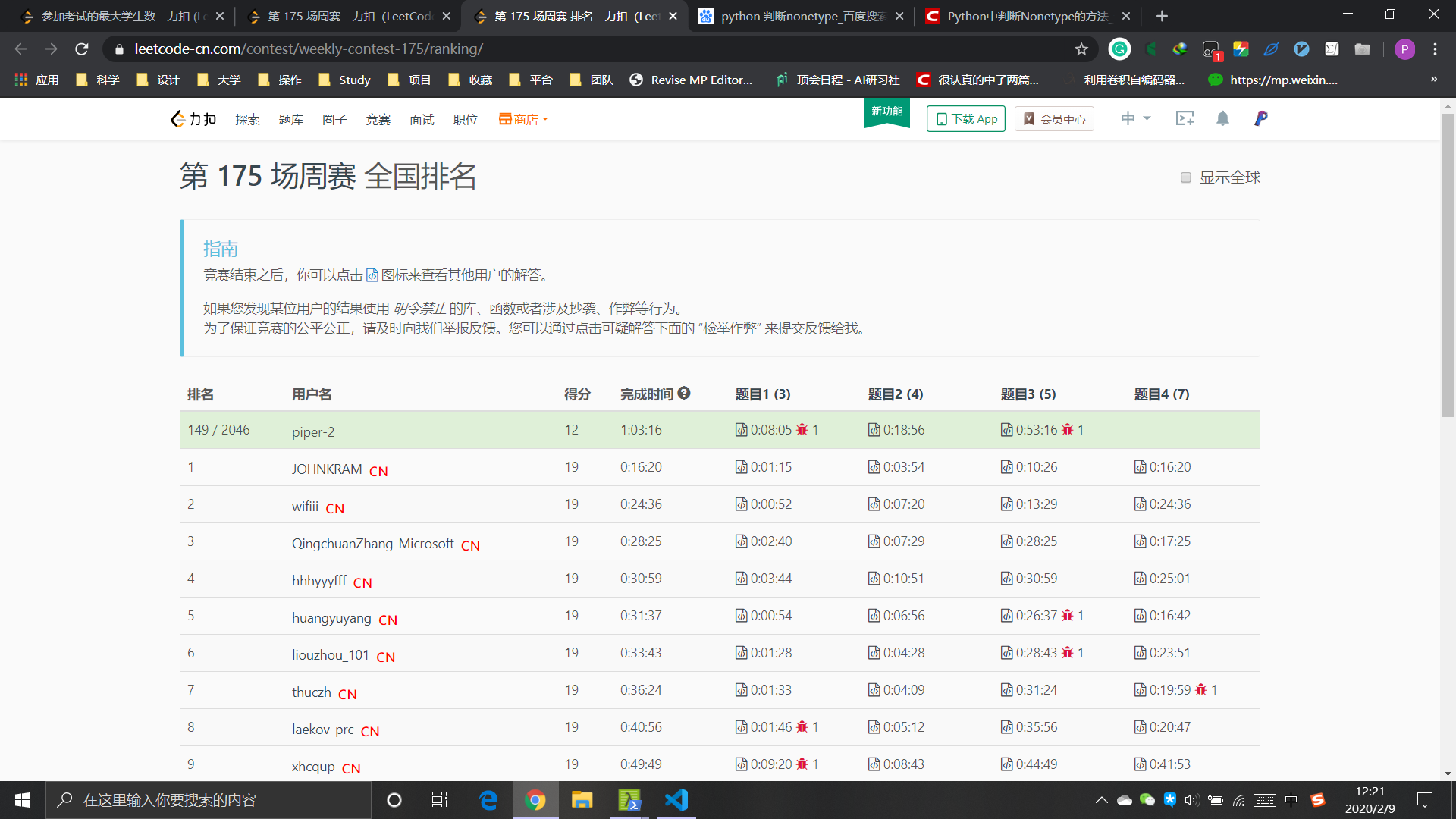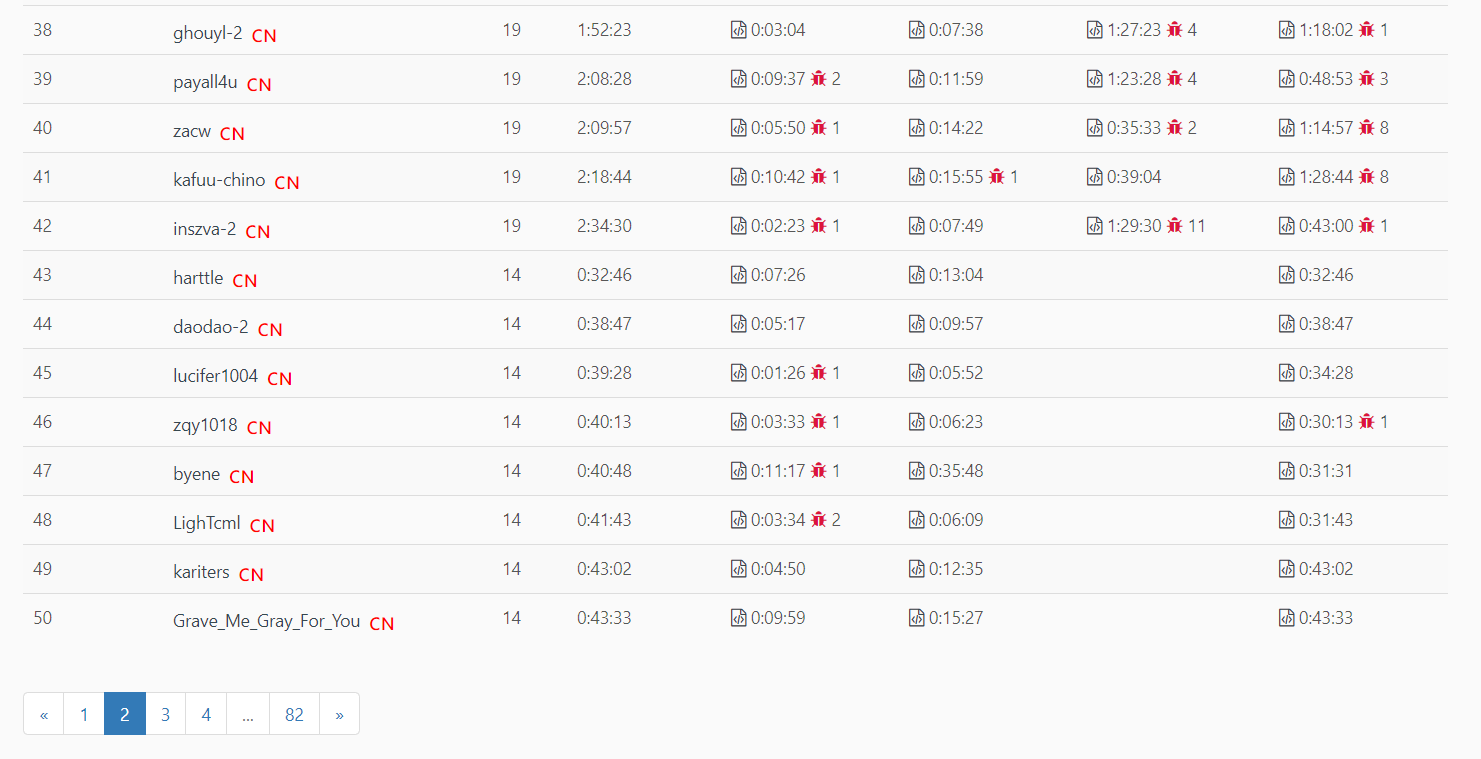+关注

2019-07(3)

2019-08(103)

2019-09(115)

2019-10(9)

2019-11(3)

2019-12(16)

2020-01(23)

2020-02(20)

## 第二次力扣周赛：排名149 / 2046；在完赛边缘打转（总结了5点，实力还不够）• 题目难度不大，竞争对手水平两极分化；
• “马虎”（将在下面的复盘中讨论什么是“马虎”，以及如何避免）；
• 起码的时间复杂度；
• 调试；
• 关掉 QQ 与微信！

#### 1. 竞争环境2046人报名参赛，只有42人完赛。竞争对手水平普遍一般。

#### 2. 复盘：“别马虎！”

##### 5332. 检查整数及其两倍数是否存在
def checkIfExist(self, arr) -> bool:
def twice(a, b):
if a == b * 2:
return True
if a * 2 == b:
return True
return False

for i in range(len(arr)):
for j in range(len(arr) - i - 1):
if twice(arr[i], arr[j+i+1]):
# if twice(arr[i], arr[j]):
return True
return False
1234567891011121314

##### 5333. 制造字母异位词的最小步骤数

def minSteps(self, s: str, t: str) -> int:
s_s = dict()
t_s = dict()
count = 0

for char in s:
s_s.setdefault(char, 0)
s_s[char] += 1

for char in t:
s_s.setdefault(char, 0)
s_s[char] -= 1
if s_s[char] < 0:
count += 1

return count
12345678910111213141516

##### 5334. 推文计数

class TweetCounts:

def __init__(self):
# (name, [time_list])
self.info = dict()

def recordTweet(self, tweetName: str, time: int) -> None:
self.info.setdefault(tweetName, list())
self.info[tweetName].append(time)

def getTweetCountsPerFrequency(self, freq: str, tweetName: str, startTime: int, endTime: int):
delta = 60
if freq == "minute":
delta = 60
if freq == "hour":
delta = 60 * 60
if freq == "day":
delta = 60 * 60 * 24
rts = list()
import math
count = math.ceil((endTime - startTime + 1) / delta)
count = max(1, count)
time_list = self.info[tweetName]
for i in range(count):
start = startTime + i * delta
end = min(start + delta, endTime + 1)
c = 0
for time in time_list:
if start <= time < end:
c += 1
rts.append(c)
if len(rts) == 0:
return 0
return rts

# Your TweetCounts object will be instantiated and called as such:
# obj = TweetCounts()
# obj.recordTweet(tweetName,time)
# param_2 = obj.getTweetCountsPerFrequency(freq,tweetName,startTime,endTime)
123456789101112131415161718192021222324252627282930313233343536373839

# [(name, time)]
self.info = list()
# search
for n, t in self.info:
if n == tweetName and start_time <= t <= end_time:
rts += 1
123456

• 尽管逻辑上没有错误，但是查找每个用户，都需要对所有 info 进行查找，浪费时间；
• 因此提交代码后，报错（大量数据下，运行超时）。

# (name, [time_list])
self.info = dict()
# search
for t in self.info[tweetName]:
if start_time <= t <= end_time:
rts += 1
123456

##### 5335. 参加考试的最大学生数

BFS 可以用递归来写，但这着实花了我一些时间。我的解决方案如下。

def maxStudents(self, seats) -> int:
xL = len(seats)
yL = len(seats)
useable = list()
for i in range(xL):
for j in range(yL):
if seats[i][j] != '#':
useable.append((j, i))

def ok(a, b):
if a - 1 == b and (a == b or a + 1 == b):
return False
if a + 1 == b and (a == b or a + 1 == b):
return False
if b + 1 == a and (b == a or b + 1 == a):
return False
if b - 1 == a and (b == a or b + 1 == a):
return False
return True

rts = set()
def bfs(seat, used_list, notused_list, count):

if notused_list is None:
return
used_list = used_list.copy()
notused_list = notused_list.copy()
for nextSeat in notused_list:
seat_ok = True
for s in used_list:
if not ok(s, nextSeat):
seat_ok = False
if seat_ok:
count += 1
used_list.append(nextSeat)
notused_list.remove(nextSeat)
bfs(nextSeat, used_list, notused_list, count)
return

for s in useable:
tmp = useable.copy()
tmp.remove(s)
bfs(s, [s], tmp, 1)
return max(rts)
12345678910111213141516171819202122232425262728293031323334353637383940414243444546

[[".",".","#","#",".","#","#"],["#","#","#",".","#","#","."],
[".","#",".","#",".","#","."],[".","#",".","#",".",".","#"],
[".",".","#","#",".","#","."],["#",".","#","#",".","#","#"]]
123

#### 3. 比赛经验：调试环境与屏蔽干扰！

class Solution:
def checkIfExist(self, arr) -> bool:
...

solution = Solution()
arr = test_data_1
arr = test_data_2
# arr = test_data_3
print(solution.checkIfExist(arr))
123456789

PiperLiu
2020-2-9 15:28:14

3 0

#### 优质资源排行榜

python经典电子书大合集下载 下载次数 8138

零基础大数据全套视频400G 下载次数 7006

零基础软件测试全套系统教程 下载次数 6506

全套人工智能视频+pdf 下载次数 6443

IOS全套视频教程 基础班+就业班 下载次数 4680

编程小白的第一本python入门书（高清版）PDF下载 下载次数 3647

11  Python深度学习 pdf下载 下载次数 3156

12  笨办法学python pdf下载 下载次数 3087

14  树莓派Python编程指南 pdf下载 下载次数 3011

16  python项目开发视频 下载次数 3002

18  黑马2017年java就业班全套视频教程 下载次数 2992

19  Python基础教程 pdf下载 下载次数 2988

21  利用python实现程序内存监控脚本 下载次数 2987

22  老男孩python自动化视频 下载次数 2983

23  老王python基础+进阶+项目视频教程 下载次数 2974

24  尚硅谷Go学科全套视频 下载次数 2972

25  某硅谷Python项目+AI课程+核心基础视频教程 下载次数 2968

26  Web前端实战精品课程 下载次数 2967

27  Python算法教程_中文版 pdf下载 下载次数 2966

28  tron python小游戏 下载次数 2963

29  [小甲鱼]零基础入门学习Python 下载次数 2962

30  老男孩python全栈开发15期 下载次数 2958

31  2017最新web前端开发完整视频教程附源码 下载次数 2948

32  最新全套完整JAVAWEB2018开发视频 下载次数 2926

33  流畅的Python PDF下载高清完整扫描原版 下载次数 2919

34  Spring boot实战视频6套下载 下载次数 2910

35  python全套视频十五期（116G） 下载次数 2908

36  Python高性能编程 pdf下载 下载次数 2906

37  Python项目实战 下载次数 2887

38  30个小时搞定Python网络爬虫 含源码 下载次数 2884

40  利用Python进行数据分析 pdf下载 下载次数 2884

41  python全自动抢火车票教程-python视频教程下载 下载次数 2883

43  Python A~B~C~ python视频教程下载 下载次数 2866

44  数据结构与算法视频（小甲鱼讲解-全） 下载次数 2864

45  web小程序表白天数倒计时源码下载 下载次数 2863

46  python基础视频教程 下载次数 2862

47  go语言全套视频 下载次数 2855

48  清华学霸尹成Python爬虫视频-ok 下载次数 2846

49  黑马前端36期最全视频和代码 下载次数 2843

50  2018最新全套web前端视频教程+源码下载 下载次数 2841